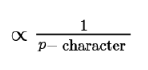Question:

The correct statement about $\mathrm{B}_{2} \mathrm{H}_{6}$ is:

1.  All B-H-B angles are of $120^{\circ}$.

2. Its fragment, $\mathrm{BH}_{3}$, behaves as a Lewis base.

3. Terminal B-H bonds have less p-character when compared to bridging bonds.

4.  The two $\mathrm{B}-\mathrm{H}-\mathrm{B}$ bonds are not of same length.

Correct Option: , 3

Solution:

Terminal bond angle is greater than that of bridge bond angle Bond angle

Bond order $\propto$ S-character